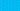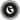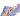PricingResources

# What is the break even point?Written by

Once you’ve invested money in a new business, one of the first questions you might be asking is when does that investment turn to profit? Performing a break even analysis helps give you some idea of when your project will pass the break even point to become profitable.

## Understanding the break even point

The break even point simply refers to the stage when a business’s revenues become equal to its costs. It can be calculated in two ways:

• Units that need to be sold until the company breaks even

• Monetary figure representing the sales needed to break even

When current revenues are falling below the break even point, this means your company is operating at a loss. As a result, it’s used as a risk indicator and metric of sustainability.

## Purpose of a break even point analysis

The break even point figure can be used as a guide for tweaking production levels or increasing a sales drive. Break even analysis can be performed by a start-up during the initial planning stages, or by existing businesses before launching a new service.

This metric isn’t typically used by outside parties like financial institutions or investors. However, while an investor might not look at the break even point for a particular company, the same calculations may be used for their own investment activities.

## How to calculate break even point

There are two methods to choose from when determining how to calculate break even point. The first is to divide the total fixed costs by revenue expressed in units. This shows how many units must be sold to break even. The second option is to divide total fixed costs by sales price, which shows the level of revenue expressed in sales, rather than unit.

## Break even point formula

The best break even point formula to use will depend on whether you decide to calculate the figure in units or sales revenue. Businesses that sell physical products might prefer the first option, while those selling services might prefer the second.

To calculate break even point using units:

By contrast, to calculate break even point using sales in GBP, you must first find the contribution margin. This is calculated by subtracting the variable costs from the product’s price and dividing it by the sale price per unit:

Therefore, in this case, the break even point formula would look like this:

For the purposes of these formulas, fixed costs refer to any costs that stand separately from the number of items sold. This could include advertising fees, office rental fees, and production costs. Variable costs refer to the amount of money paid for items like parts and labour, which can be impacted by fluctuations in the market.

## Break even point example

To illustrate how this type of calculation works, imagine the following real-world break even point example. A small batch ice cream company is considering the launch of a new peppermint flavour. The company needs to know its break even point to determine whether the product will be worth investing in. For the first month of production, the costs would be:

• Fixed Costs = £3,000

• Variable Costs = £0.50 (per small container of ice cream)

• Sales Price = £2.50 (per small container)

To calculate the break even point per unit, the formula would be:

• £3000 / (2.50 – 0.50) or 3000 / 2.0

This would equal 1500 units, meaning that the ice cream company would need to sell 1500 containers of its new peppermint flavour in the first month to break even.

Expressed in GBP, you must first calculate the contribution margin with:

• (2.50 – 0.50) / 2.50 = 0.8

Then, plug this number into the break even point formula:

• £3000 / 0.8 = £3,750

This means that the ice cream company would need to sell £3,750 of the new peppermint flavour to break even. With this information in hand, the company can think about whether these sales figures are achievable in the current market conditions.

## We can help

GoCardless helps you automate payment collection, cutting down on the amount of admin your team needs to deal with when chasing invoices. Find out how GoCardless can help you with ad hoc payments or recurring payments.Contact sales

Sales

Support